# Optimizing Basic Power Measurements on NI Vector Signal Analyzers

## Overview

Measuring the power of an RF signal is key to characterizing and testing RF components and devices. Part of measuring power accurately is possessing a firm understanding of the characteristics of power and how it correlates to the time and frequency domain representations of a signal. This article will explain two key settings, reference level and resolution bandwidth (RBW), and how they effect a power measurement. Note that there are many different methods and types of equipment that can be used to measure power. There are three primary equipment types in the RF industry for doing power analysis: power meters, spectrum analyzers, and vector signal analyzers. In this article, we will be approaching these topics from the perspective of power as measured by a Vector Signal Analyzer (VSA), more specifically using the NI VSA hardware and NI-RFSA driver settings for illustration.

## Time domain vs. Frequency Domain Power

Let’s begin by looking at the time domain power envelope of a standard GSM burst. GSM bursts employ a GMSK modulation scheme, which lend themselves well to a basic discussion of power analysis since their power profiles are constant within the active period of the burst.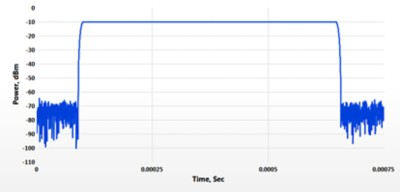Figure 1. Power vs. Time of a GSM signal

In Figure 1, notice the PvT plot is not a complete representation of the GSM signal itself, but a representation of the envelope power as measured through a bandpass filter across a period of time. For the GSM burst in Figure 1, the power in the active portion of the burst is -10 dBm.

Now compare Figure 1 with the corresponding spectrum associated with the same signal in Figure 2. While the power is constant, much like a Continuous Wave (CW), the signal occupies some non-zero bandwidth in frequency. The bandwidth of the signal is usually declared as a percentage of total power acquired, since the bandwidth of the GSM signal is technically infinite.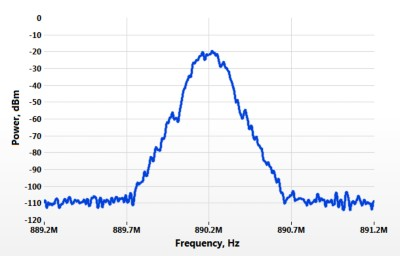Figure 2. Spectrum of a GSM signal (10k RBW, Ref. Level -10dBm)

We’ll return to bandwidth later in the article. Let’s get back to understanding the analysis of power in both the time and frequency domains. We can see some details of the power of the signal by observing Figure 1. At a high level, the spectrum is a frequency domain representation of the signal acquired by the analyzer over a period of time. It shows how power is distributed across a frequency span for a given signal. In regards to Figure 1, integrating the power over the channel bandwidth in the frequency domain should result in the same -10 dBm power level observed, contrary to the common mistake of declaring the peak of the spectral trace (-20dBm) as the maximum power of the signal.

To understand this better, consider the simple discrete spectrum in Figure 3. Each point shows the power over 1MHz frequency bands. A spectrum is simply a series of smaller bandwidth power measurements at specific frequencies stitched together.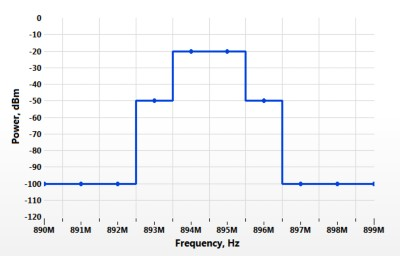Figure 3. Simple example spectrum showing power discretized into frequency bins

To determine the total power in this frequency range, we need to integrate the power in each bandwidth over the span. In order to do this, we cannot simply add the decibel values -- we must convert to linear units first, then sum, and then convert back to decibels. The dBm conversion equation is below for reference:

P(dBm) = 10*log(P(mw)/1mW)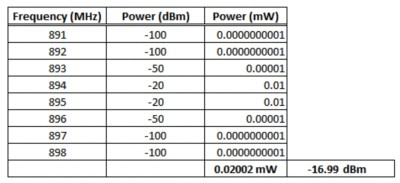Adding up the power in Watts from the table above gives approximately 20 mW. Now convert that to dBm as shown below using the equation above. The power at 893M and 896M are -30dB from the primary power at 894M and 895M, and contribute a negligible amount of power to the overall signal.

### Fundamental Settings for Basic Power Measurements

The following sections will use the same GSM waveform example to discuss reference level and resolution bandwidth for RF power measurements using a VSA or spectrum analyzer.

## Reference Level

In the NI-RFSA driver, the Configure Reference Level VI is used to set the reference level of the VSA. This must be set before calling the niRFSA Initiate VI.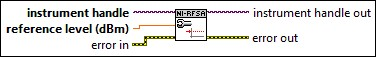Figure 4. Screenshot of niRFSA Configure Reference Level VI from NI-RFSA Help documentation

The reference level setting tells the analyzer the expected RMS power of all signals entering the analyzer. This is an important measurement setting for a couple of reasons. First, it adjusts gain and attenuation stages in the signal path to reduce compression in the amplification stages and prevent damage to the device. Second, the driver attempts to optimize its gain and attenuation settings in order to minimize noise in the measurement. Hence, it’s important to understand the nature of the input signal and to choose an appropriate reference level for the instrument.

Recall from the PvT graph in Figure 1 that the maximum RMS power was around -10 dBm. It’s clear by observing Figure 5 the reference level should be set at or above -10 dBm to ensure the analyzer is operating well within its linear range.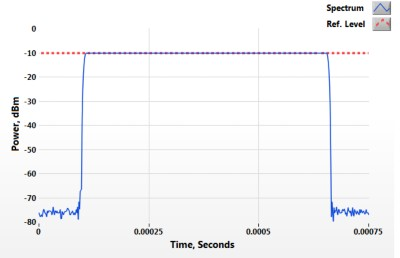Figure 5. Power vs. Time of a GSM signal with the reference level

For comparison, look at the reference level as it relates to the corresponding spectrum shown in Figure 6. Note that the reference level is about 10 dB higher than the peak point along the spectrum trace. This is due to the fact that the spectrum shows data separated in frequency as opposed to the actual integrated power of the entire signal itself, as discussed in the previous section.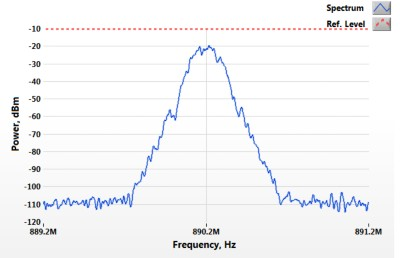Figure 6. GSM Spectrum with reference level (10 kHz RBW, Ref. Level -10 dBm)

### Reference Level Too High

Consider the implications in the time domain. See Figure 7 below, showing a GSM signal acquired with an appropriate reference level next to the same signal measured with a higher reference level. Notice that the measurement is noisier with a higher reference level. This is because the RF attenuation is too high and pushes the signal closer to the analyzer’s noise floor and decreases the signal to noise ratio of the measurement.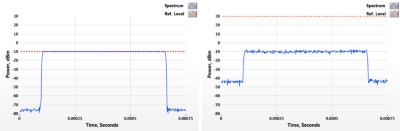Figure 7. Left: GSM with an appropriate reference level. Right: GSM with a reference level too high.

Now consider the frequency domain shown in Figure 8. The increase in the noise floor is even more apparent, making it harder to see the full shape of the GSM channel. If the signal level were any lower, it would be overcome by the noise floor due to excessive RF attenuation.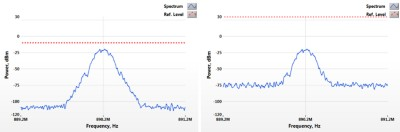Figure 8. Left: GSM signal with appropriate reference level. Right: GSM with reference level too high.

### Reference Level Too Low

Consider Figure 9 below, which compares Figure 1 next to the same signal measured with a reference level that’s too low. A reference level set too low causes compression that can be seen in the time domain. Since there is less RF attenuation for the same power signal, the amplifiers are driven into compression.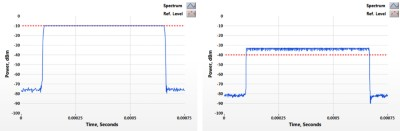Figure 9. Left: GSM signal Power vs. Time with a -10 dBm reference level. Right: GSM signal Power vs. Time with -40 dBm reference level.

Setting the reference level too low may result in damage to the device. For this reason, the driver will warn the user if it detects power too high above the expected reference level, or throw an error if it is too low.

Now consider Figure 8 showing the frequency domain. The spectrum is now distorted due to the compression observed in the PvT plots from Figure 7. The distortion creates additional frequency content that causes the noise floor to increase.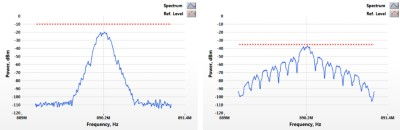Figure 10. Left: GSM spectrum with an appropriate reference level. Right: GSM spectrum with a reference level set too low.

Above, we discussed how adjusting the reference level too high or too low can affect a power measurement. There are some other tradeoffs to consider. For instance, consider a signal whose power is very close to the noise floor. Averaging multiple acquisitions can be used to drop the displayed noise floor slightly to resolve the signal. While this allows the signal to be measured, it also increases the total measurement time due to the additional acquisitions required for averaging.

## Resolution Bandwidth (RBW)

In the NI-RFSA driver, the resolution bandwidth is set using the niRFSA Configure Resolution Bandwidth VI. It must be set before niRFSA Initiate VI is called.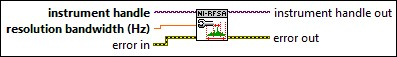Figure 11. Screenshot of niRFSA Configure Resolution Bandwidth VI taken from NI-RFSA Help documentation

It’s worth briefly discussing resolution bandwidth as it relates to traditional swept-tuned spectrum analyzers. In swept-tuned analyzers, the RBW filter is implemented in hardware with a power sensor directly following it. Depending on the RBW and span that is configured, the signal is stepped through the filter in frequency to capture the average power in the frequency window. The average power in each step is then plotted on the spectrum. For this reason, a smaller RBW causes the measurement to take longer since the signal must be moved multiple times to cover the entire configured measurement span. In addition, the signal must wait in each step long enough to overcome the delay inherent in the filter, which also adds time to the measurement.

National Instruments Vector Signal Analyzers are FFT-based as opposed to the swept-tuned analyzers discussed above. The main difference is that instead of relying on hardware to move a signal through a bandpass RBW filter for frequency selective power analysis, the RBW filter is implemented in software through the Fast Fourier Transform. One key benefit to this is that analyzing small frequency spans is faster. In addition, FFT-based analyzers also have the ability to measure phase, which enables them to perform demodulation.

Most commonly, RBW is thought of as a way to control the resolution of the spectrum. This however, is only half of the story. Consider the implications in the time domain, say for a PvT measurement. Recall Figure 1 and how the PvT measures the average power of the signal as measured through a resolution bandwidth (RBW) filter at any given point in time. Let’s see what happens when we push the RBW to the extremes and discuss the implications in both the time and frequency domains.

### Resolution Bandwidth Too High

A higher RBW implies an acquisition with a larger number of points. For this reason, a RBW too large can cause system acquisition memory errors. With a larger number of points, this increases the signal processing time. As well, the power of the noise floor increases since the filter is wider and letting more noise through.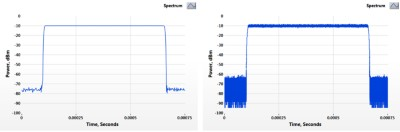Figure 12. Left: GSM Power vs. Time with 1 MHz RBW. Right: GSM Power vs. Time with 80 MHz RBW.

In the spectrum, there’s little to no resolution or definition to the shape of the signal. It’s hard to distinguish the channel bandwidth from the noise floor in this case.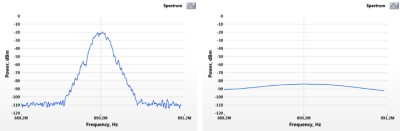Figure 13. Left: GSM Spectrum acquired with 10kHz RBW. Right: GSM Spectrum acquired with 1 MHz RBW.

### Resolution Bandwidth Too Low

A lower RBW implies a longer acquisition time with fewer points per step. As such, a RBW too small may not allow key signal characteristics to pass through, causing them to be missed. This is especially true for burst signals that are only active for a short amount of time wherein the frequency content of interest is located.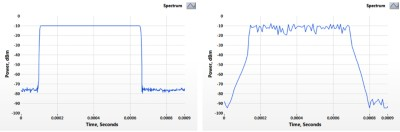Figure 14. Left: GSM Power vs. Time acquired with 1 MHz RBW. Right: GSM Power vs. Time acquired with 100 kHz RBW.

Notice how there is more resolution when looking at the corresponding spectrum shown in Figure 15. In this case, the bandwidth of the GSM signal is more pronounced and easily seen, however the power is inaccurate due to the RBW filter not being wide enough to capture the entire signal's bandwidth.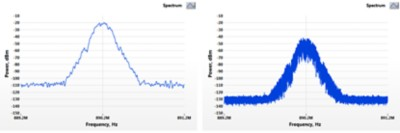Figure 15. Left: GSM Spectrum acquired with 10 kHz RBW. Right: GSM spectrum acquired with 100 Hz RBW.

When it comes to choosing the right RBW, it depends on the measurement and the type of signal that is being measured. For bursted signals, the RBW must be high enough to capture the entire signal’s bandwidth, but not so high that the noise floor becomes too large.

RBW also affects measurement time. A smaller RBW increases the measurement time due to the increased number of RBW points needed to complete the spectrum.

## Conclusion

This document provided an introduction into how to choose an appropriate reference level and RBW and the consequences of choosing a value too high or too low for the measurement. The implications of this were illustrated with an example GSM signal. Reference level and RBW are basic settings that can have a noticeable impact on a power measurement, and it’s important to be able to apply some intuition when configuring and analyzing RF power measurements.

While this article focused on National Instruments Vector Signal Analyzers, the concepts may apply to other VSAs as well. This article should encourage engineers to investigate other configurations and how they can have an effect on their RF measurements.